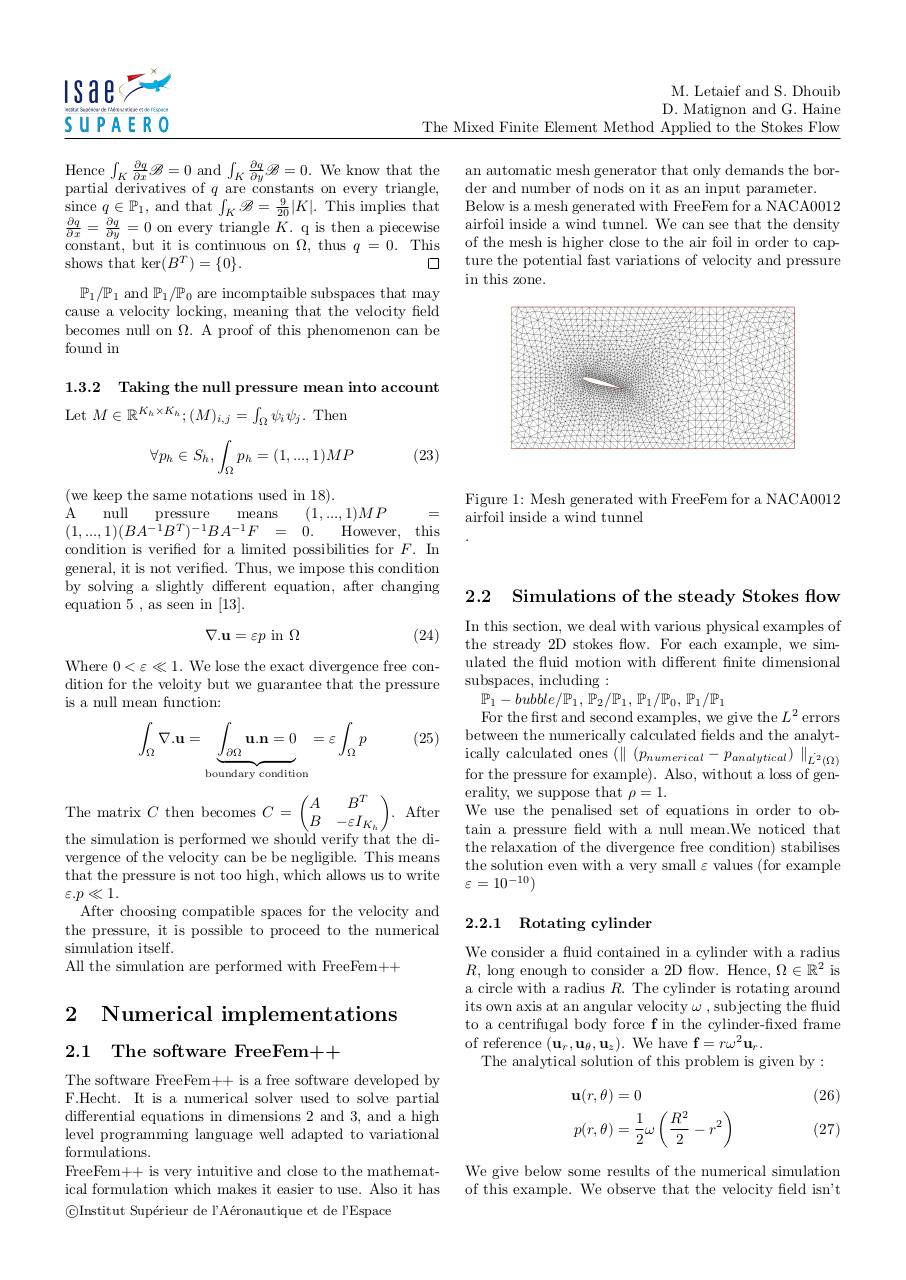# rapport (1).pdfPage 1 2 3 4 5 6 7 8

#### Text preview

M. Letaief and S. Dhouib
D. Matignon and G. Haine
The Mixed Finite Element Method Applied to the Stokes Flow
R ∂q
R ∂q
Hence K ∂x
B = 0 and K ∂y
B = 0. We know that the
partial derivatives of qRare constants on every triangle,
9
|K|. This implies that
since q ∈ P1 , and that K B = 20
∂q
∂q
∂x = ∂y = 0 on every triangle K. q is then a piecewise
constant, but it is continuous on Ω, thus q = 0. This
shows that ker(B T ) = {0}.

an automatic mesh generator that only demands the border and number of nods on it as an input parameter.
Below is a mesh generated with FreeFem for a NACA0012
airfoil inside a wind tunnel. We can see that the density
of the mesh is higher close to the air foil in order to capture the potential fast variations of velocity and pressure
in this zone.

P1 /P1 and P1 /P0 are incomptaible subspaces that may
cause a velocity locking, meaning that the velocity field
becomes null on Ω. A proof of this phenomenon can be
found in
1.3.2

Taking the null pressure mean into account
R
Let M ∈ RKh ×Kh ; (M )i,j = Ω ψi ψj . Then
Z
∀ph ∈ Sh ,
ph = (1, ..., 1)M P
(23)

(we keep the same notations used in 18).
A
null
pressure
means
(1, ..., 1)M P
=
(1, ..., 1)(BA−1 B T )−1 BA−1 F = 0.
However, this
condition is verified for a limited possibilities for F . In
general, it is not verified. Thus, we impose this condition
by solving a slightly different equation, after changing
equation 5 , as seen in .
∇.u = εp in Ω

(24)

Where 0 &lt; ε  1. We lose the exact divergence free condition for the veloity but we guarantee that the pressure
is a null mean function:
Z
Z
Z
∇.u =
u.n = 0 = ε
p
(25)

}
| ∂Ω {z
boundary condition


A
BT
The matrix C then becomes C =
. After
B −εIKh
the simulation is performed we should verify that the divergence of the velocity can be be negligible. This means
that the pressure is not too high, which allows us to write
ε.p  1.
After choosing compatible spaces for the velocity and
the pressure, it is possible to proceed to the numerical
simulation itself.
All the simulation are performed with FreeFem++


2
2.1

Numerical implementations
The software FreeFem++

The software FreeFem++ is a free software developed by
F.Hecht. It is a numerical solver used to solve partial
differential equations in dimensions 2 and 3, and a high
level programming language well adapted to variational
formulations.
FreeFem++ is very intuitive and close to the mathematical formulation which makes it easier to use. Also it has
c
Institut
Sup´erieur de l’A´eronautique et de l’Espace

Figure 1: Mesh generated with FreeFem for a NACA0012
airfoil inside a wind tunnel
.

2.2

Simulations of the steady Stokes flow

In this section, we deal with various physical examples of
the stready 2D stokes flow. For each example, we simulated the fluid motion with different finite dimensional
subspaces, including :
P1 − bubble/P1 , P2 /P1 , P1 /P0 , P1 /P1
For the first and second examples, we give the L2 errors
between the numerically calculated fields and the analytically calculated ones (k (pnumerical − panalytical ) kL˙2 (Ω)
for the pressure for example). Also, without a loss of generality, we suppose that ρ = 1.
We use the penalised set of equations in order to obtain a pressure field with a null mean.We noticed that
the relaxation of the divergence free condition) stabilises
the solution even with a very small ε values (for example
ε = 10−10 )
2.2.1

Rotating cylinder

We consider a fluid contained in a cylinder with a radius
R, long enough to consider a 2D flow. Hence, Ω ∈ R2 is
a circle with a radius R. The cylinder is rotating around
its own axis at an angular velocity ω , subjecting the fluid
to a centrifugal body force f in the cylinder-fixed frame
of reference (ur , uθ , uz ). We have f = rω 2 ur .
The analytical solution of this problem is given by :
u(r, θ) = 0
p(r, θ) =

1
ω
2

(26)


2

R
− r2
2


(27)

We give below some results of the numerical simulation
of this example. We observe that the velocity field isn’t